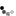# Differential Amplifier - Large Input Signal

A differential amplifier is a type of electronic amplifier that amplifies the difference between two input voltages only. The differential amplifier two inputs are the inverting input and non-inverting input. Its output signal is 180° out of phase with inverting input signal and in phase with non-inverting input signal.

The different modes of operation of the differential amplifier are:

• Common mode input signal vCM. Both inputs are connected together to the input signal.
• Large differential input signal.
• Small differential input signal vi<2vT

The circuit below shows the differential amplifier with a large differential input signal. When an Operational Amplifier (OPAMP) is used in this configuration it is also called a comparator.Notes:

• When both V1 and V2 are 2.5V, see the small signal input circuit DC analysis for calculating the voltages.
• When V2-2vT < V1 < V2+2vT, the small signal input circuit AC analysis applies.
• When V1>V2+2vT, Q1 is on and Q2 is off. \begin{equation} V_1 = V_{R1} + V_{BE} \end{equation} Ignoring IB, thus IC = IE \begin{equation} V_{R2} = {(V_1 - V_{BE})R2 \over R1} \end{equation}
• When V1<V2-2vT, Q1 is off and Q2 is on. \begin{equation} V_2 = V_{R1} + V_{BE} \end{equation} \begin{equation} V_{R3} = {(V_2 - V_{BE})R3 \over R1} \end{equation}
• Move the knob to see the effects of V1 on the inverting and non-inverting outputs.